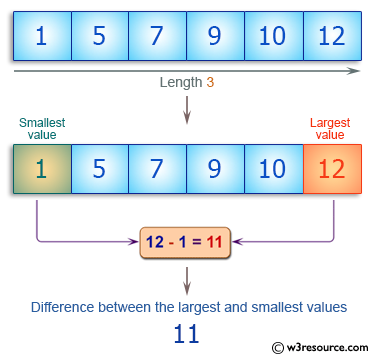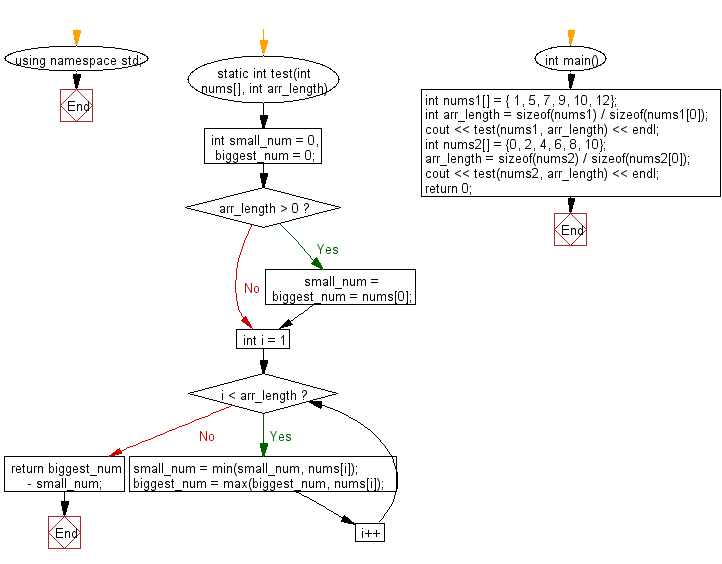﻿ C++ : Difference, largest and smallest value in an array# C++ Exercises: Compute the difference between the largest and smallest values in a given array of integers and length one or more

## C++ Basic Algorithm: Exercise-99 with Solution

Write a C++ program to compute the difference between the largest and smallest values in a given array of integers and length one or more.

Sample Solution:

C++ Code :

``````#include <iostream>
using namespace std;

static int test(int nums[], int arr_length)
{
int small_num = 0, biggest_num = 0;

if (arr_length > 0) small_num = biggest_num = nums;

for (int i = 1; i < arr_length; i++)
{
small_num = min(small_num, nums[i]);
biggest_num = max(biggest_num, nums[i]);
}

return biggest_num - small_num;
}

int main()
{
int nums1[] = { 1, 5, 7, 9, 10, 12};
int arr_length = sizeof(nums1) / sizeof(nums1);
cout << test(nums1, arr_length) << endl;
int nums2[] = {0, 2, 4, 6, 8, 10};
arr_length = sizeof(nums2) / sizeof(nums2);
cout << test(nums2, arr_length) << endl;
return 0;
}
``````

Sample Output:

```11
10
```

Pictorial Presentation:Flowchart:C++ Code Editor: